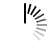# Tower Of Hanoi Problem

Tower of Hanoi is a puzzle problem. Where we have three stands and n discs. Initially, Discs are placed in the first stand. We have to place discs into the third or destination stand, the second or auxiliary stand can be used as a helping stand.

1. But there are some rules to follow.
2.  We can transfer only one disc for each movement.
3. Only the topmost disc can be picked up from a stand.
4. No bigger disc will be placed at the top of the smaller disc.

This problem can be solved easily by recursion. At first, using recursion the top (n-1) discs are placed from source to auxiliary stand, then place the last disc from source to destination, then again place (n-1) disc from auxiliary stand to destination stand by recursion.

#### Input and Output

```Input:
Number of discs: 3
Output:
1. Move disk 1 from A to C
2. Move disk 2 from A to B
3. Move disk 1 from C to B
4. Move disk 3 from A to C
5. Move disk 1 from B to A
6. Move disk 2 from B to C
7. Move disk 1 from A to C```

#### Algorithm

`toh(n, s, a, d)`

Input: Number of discs, source, auxiliary, destination.

Output: Steps to move discs from source to destination maintaining proper rules.

```Begin
if n = 1, then
display move disc from s to d
toh(n-1, s, d, a)

display move disc from s to d
toh(n-1, a, s, d)
End```

#### Source Code (C++)

```#include<iostream>
using namespace std;

void TOH(int n, char s, char a, char d) {
static int count = 0;          //store number of counts
if(n == 1) {
count++;
cout << count<< ". Move disk " << n << " from "<<s <<" to "<<d<<endl;
return;      //base case, when only one disk
}

TOH(n-1, s, d, a);               //recursive call the function
count++;
cout << count<< ". Move disk " << n << " from "<<s <<" to"<<d<<endl;
TOH(n-1, a, s, d);
}

int main() {
int n;
cout << "Enter the number of disks: ";
cin >> n;
TOH(n, 'A','B','C');
}```

#### Output

```Enter the number of disks: 3
1. Move disk 1 from A to C
2. Move disk 2 from A to B
3. Move disk 1 from C to B
4. Move disk 3 from A to C
5. Move disk 1 from B to A
6. Move disk 2 from B to C
7. Move disk 1 from A to C```